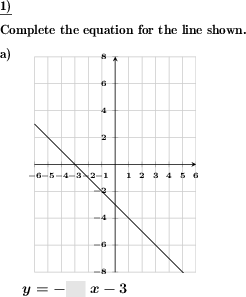Custom math worksheets at your fingertips# Details for problem "Complete equation for given straight line"

Quickname: 9005

Elementary School, Primary School, Junior High School, Middle School, High School.

## Summary

In a straight line equation for a given line, gaps must be filled in correctly.

## Example## Description

Parts of the equation for a straight line are given. The missing parts have to be filled it. The corresponding straight line is given in a coordinate system, the slope and Y-axis intercept can be read off there.

The straight line equation and the dimensions of the coordinate system are designed in such a way that the rise and run can be read without particular difficulties. The Y-axis intercept, for example, can be selected to lie on only full or even half coordinate plane squares (vertical divisions). It is preselectable whether the straight line may, may not or even must pass through the origin.

With regard to the coordinate system, it can be set whether only quadrant one is to be used with all positive X and Y coordinates, or also the quadrants containing coordinates with negative signs. The coordinate system can be output in three different sizes.

The slope is always such that a slope triangle would be a maximum of four boxes wide, i.e. would have a divisor of maximum four. It can be limited to only positive or only negative slope, with or without the possibility of a slope of zero.

Which parts of the straight line equation are to be completed can be specified as follows:

• m (slope) and b (y axis intercept)
• m only
• b only
• m and b, with signs of m and b given
• m only, with sign of m given
• b only, with sign of b given
• random choice of the above
• random choice of the above, with the exception of option one, i.e. either m or bi will be given

Download free printable worksheets for this math problem here. The worksheet contains the problems only, the solution sheet includes the answers. Just click on the respective link.

•Worksheet 1Solution sheet with answers
•Worksheet 2Solution sheet with answers
•Worksheet 3Solution sheet with answers

If you can not see the solution sheets for download, they may be filtered out by an ad blocker that you may have installed. If this is the case, please allow ads for this page and reload the page. The solution sheets will then reappear.

• Do these sample worksheets do not really fit?
• Do you need more math worksheets, with a different level of difficulty?
• Would you like to combine different problems on a worksheet and adjust them to your needs?
• As a teacher, you can put together your own worksheets using the automatically generated math problems provided.
With a free initial credit, you can start creating your own math worksheets in a few minutes.

You can try it for free! Register here, to create custom worksheets now!

## Customization options for this problem

Parameter
Possible values
Number of problems
1, 2
Yes, No
Through origin
may, always, never
Slope
pos&neg or null, pos&neg not null, positive, negative
Sub-square rises
Yes, No
Size
small, medium, large
m and b are
fraction, decimal
Fill in
m and b, m, b, m, b, their signs given, b, sign of b given, m, sign of m given, random, random not (m and b)

## Similar problems

Remark
Description
Slope triangle given
The slope shall be derived from a straight line with a gradient triangle in the coordinate system.
Draw slope triangle for given line
In a coordinate system with a given straight line a slope triangle is to be drawn.
Without any hints
For a given straight line on a coordinate plane, the corresponding equation must be specified.